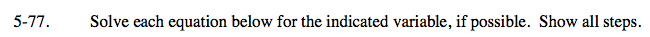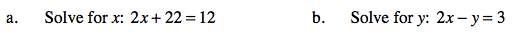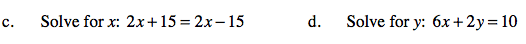Home > AC > Chapter 5 > Lesson 5.2.2 > Problem5-77

5-77.
1. Solve each equation below for the indicated variable, if possible. Show all steps. Homework Help ✎

1. Solve for x: 2x + 22 = 12

2. Solve for y: 2x − y = 3

3. Solve for x: 2x + 15 = 2x − 15

4. Solve for y: 6x + 2y = 10Solve by isolating the variable on one side of the equation by subtracting 22 from both sides.
2x = 12 − 22
2x = −10

x = −5

Solve by isolating y on one side of the equation, and then simplify as much as possible.
2x = y + 3
Subtract 3 from both sides.

2x − 3 = yUse the same strategy shown in parts (a) and (b) to solve parts (c) and (d).

y = 5 − 3x